Next: Gauss' Law Up: Electricity Previous: Example 3.2: Electrostatic force between

## Example 3.3: Electric field generated by two point charges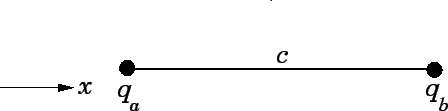Question: Two point charges,and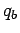, are separated by a distance. What is the electric field at a point halfway between the charges? What force would be exerted on a third charge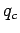placed at this point? Take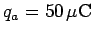,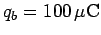,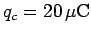, and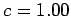m.

Solution: Suppose that the line fromtoruns along the-axis. It is clear, from Coulomb's law, that the electrostatic force exerted on any charge placed on this line is parallel to the-axis. Thus, the electric field at any point along this line must also be aligned along the-axis. Let the-coordinates of chargesandbe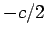and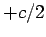, respectively. It follows that the origin (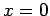) lies halfway between the two charges. The electric field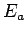generated by chargeat the origin is given by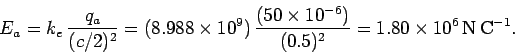The field is positive because it is directed along the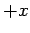-axis (i.e., from chargetowards the origin). The electric field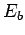generated by chargeat the origin is given by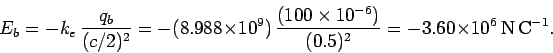The field is negative because it is directed along the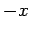-axis (i.e., from chargetowards the origin). The resultant fieldat the origin is the algebraic sum ofand(since all fields are directed along the-axis). Thus,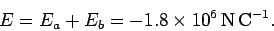Sinceis negative, the resultant field is directed along the-axis.

The force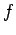acting on a chargeplaced at the origin is simply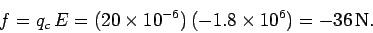Since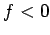, the force is directed along the-axis.Next: Gauss' Law Up: Electricity Previous: Example 3.2: Electrostatic force between
Richard Fitzpatrick 2007-07-14